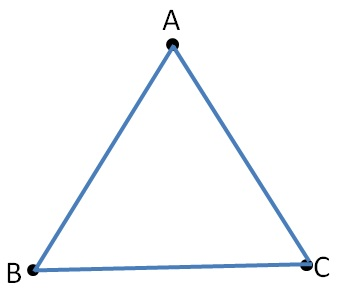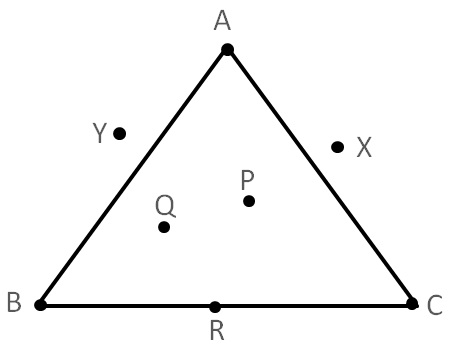Triangle is made by joining any 3 non-collinear points.

It is a 3 sided polygon.

Polygon

Polygon is a simple closed figure made of line segments

A triangle has

3 vertices − A, B, C

3 sides −     AB, BC, AC

3 angles − ∠A, ∠B, ∠C

#### Interior and Exterior of a TriangleHere,

• P, Q are in interior of ∆ABC
• R is on triangle ∆ABC
• X, Y are in exterior of ∆ABC
1. Chapter 5 Class 6 Understanding Elementary Shapes
2. Concept wise
3. Classifying triangles

Classifying triangles

Chapter 5 Class 6 Understanding Elementary Shapes
Concept wise